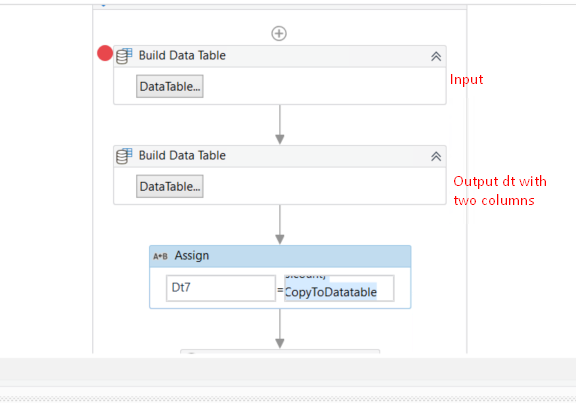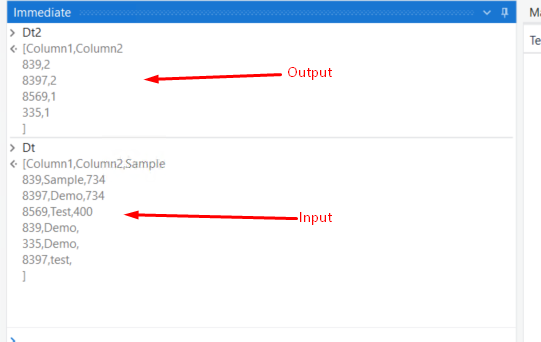# How to get count of duplicate data in a excel using LINQ query in uipath

Hi Team,

I have one excel with bulk data .
I have account number column there in excel .so, how to get count of duplicate account number in excel using LINQ query in UiPath.
The count should be int variable .

Thanks,
Baby

Hello @Baby123

You can check the below post.

Thanks

dtResult = new configured datatable with 2 cols / dtOrig clone
dtResult =

``````(From d In dtData.AsEnumerable
Group d By k= d("YourKey").toString.Trim Into grp=Group
Let c = grp.Count
Let ra = New Object(){k,c}
``````

HI @Baby123

Checkout this expression

``````(From d In Dt.AsEnumerable
Group d By row = d("Column1").ToString()
Into grp =Group
Let s=grp(0)("Column1")
Let res=New Object() {s,grp.count}